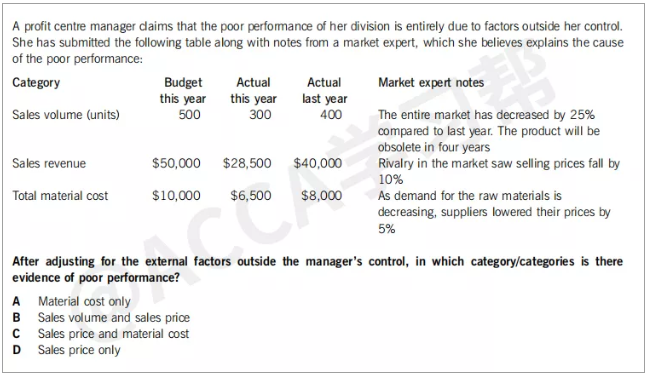PM（F5）科目中关于Variance的两道真题，你都能做对吗？

Sept 2016 No.131）Sales volume
Actual：300
Revised：看调整项，比去年下降25%，也就是400*（1-25%）=300，Actual=revised，不算好也不算坏。
2）Sales revenue
Actual:\$28,500
Revised revenue=revised sales volume*revised sales price

Original Budget的单价是\$50,000/500=\$100
100*(1-10%)=\$90

Revised revenue=\$27000&lt;actual revenue=\$28500

3）material cost
PM科目中当题目没有刻意的说销量和产量之间差异的时候，都默认产量=销量
Actual material cost=\$6500
Revised material cost=revised volume*revised material cost per unit
Revised volume=300
revised material cost per unit根据note，下降5%。
Original budget material cost per unit=\$10,000/500=\$20
revised material cost per unit=\$20*(1-5%)=\$19
Revised material cost=300*19=\$5700
Revised material cost=\$5700&gt;actual material cost=\$6500

Specimen 2016 No.5